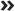ï»¿

Chapter 20 Doing More Advanced Analyses

Chapter 20

IN THIS CHAPTERConducting one-way ANOVAsRunning post hoc testsGraphing meansRunning multiple linear regressionMaking predictions

This chapter is a direct extension of Chapters 18 and 19. In Chapter 18, we discuss t-tests, which are used when the dependent variable is continuous and the independent variable has two categories. In this chapter, we will talk about the one-way ANOVA procedure, which is an extension of the independent samples t-test and is used when you have a continuous dependent variable and the independent variable has two or more categories.

In Chapter 19, we talk about simple linear regression, which is used when the dependent variable is continuous and there is one continuous independent variable. In this chapter, we talk about how to perform multiple linear regression, which is used when the dependent variable is continuous and there are various continuous independent variables. ...

Get SPSS Statistics For Dummies, 4th Edition now with the O’Reilly learning platform.

O’Reilly members experience books, live events, courses curated by job role, and more from O’Reilly and nearly 200 top publishers.